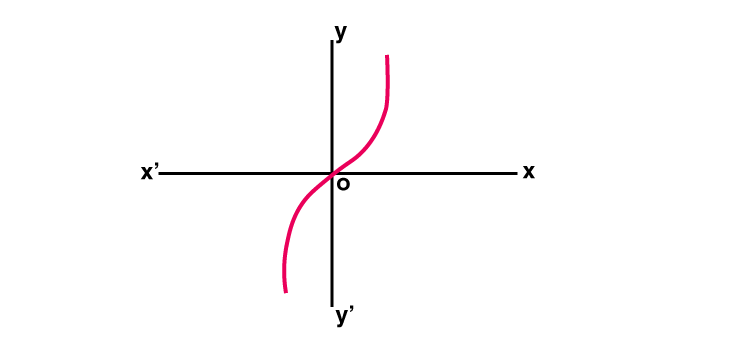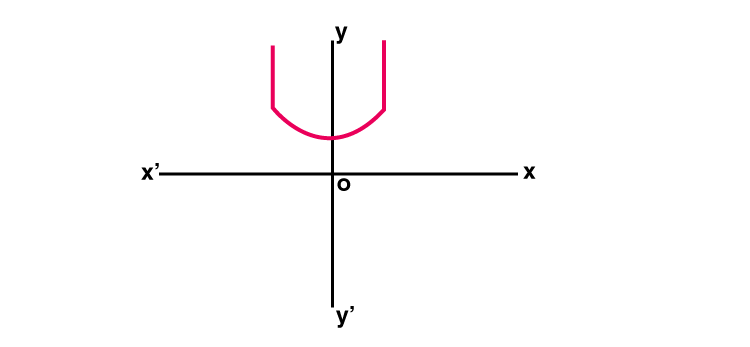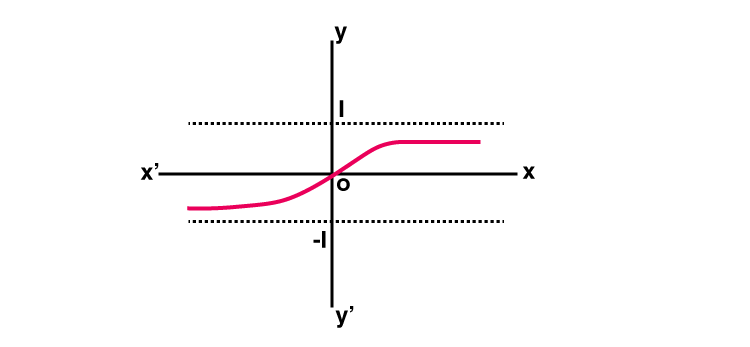# Hyperbolic Functions

In Mathematics, the hyperbolic functions are similar to the trigonometric functions or circular functions. Generally, the hyperbolic functions are defined through the algebraic expressions that include the exponential function (ex) and its inverse exponential functions (e-x), where e is the Euler’s constant. Here, we are going to discuss the basic hyperbolic functions, its properties, identities, and examples in detail.

## Hyperbolic Function Definition

The hyperbolic functions are analogs of the circular function or the trigonometric functions. The hyperbolic function occurs in the solutions of linear differential equations, calculation of distance and angles in the hyperbolic geometry, Laplace’s equations in the cartesian coordinates. Generally, the hyperbolic function takes place in the real argument called the hyperbolic angle. The basic hyperbolic functions are:

• Hyperbolic sine (sinh)
• Hyperbolic cosine (cosh)
• Hyperbolic tangent (tanh)

From these three basic functions, the other functions such as hyperbolic cosecant (cosech), hyperbolic secant(sech) and hyperbolic cotangent (coth) functions are derived. Let us discuss the basic hyperbolic functions, graphs, properties, and inverse hyperbolic functions in detail.

## Hyperbolic Functions Formulas

The basic hyperbolic functions formulas along with its graph functions are given below:

### Hyperbolic Sine Function

The hyperbolic sine function is a function f: R → R is defined by f(x) = [ex– e-x]/2 and it is denoted by sinh x

Sinh x = [ex– e-x]/2

Graph : y = Sinh x### Hyperbolic Cosine Function

The hyperbolic cosine function is a function f: R → R is defined by f(x) = [ex +e-x]/2 and it is denoted by cosh x

cosh x = [ex + e-x]/2

Graph : y = cosh x### Hyperbolic Tangent Function

The hyperbolic tangent function is a function f: R → R is defined by f(x) = [ex – e-x] / [ex + e-x] and it is denoted by tanh x

tanh x = [ex – e-x] / [ex + e-x]

Graph : y = tanh x## Properties of Hyperbolic Functions

The properties of hyperbolic functions are analogous to the trigonometric functions. Some of them are:

1. Sinh (-x) = -sinh x
2. Cosh (-x) = cosh x
3. Sinh 2x = 2 sinh x cosh x
4. Cosh 2x = cosh2x + sinh2x

The derivatives of hyperbolic functions are:

1. d/dx sinh (x) = cosh x
2. d/dx cosh (x) = sinh x

Some relations of hyperbolic function to the trigonometric function are as follows:

1. Sinh x = – i sin(ix)
2. Cosh x = cos (ix)
3. Tanh x = -i tan(ix)

## Hyperbolic Function Identities

The hyperbolic function identities are similar to the trigonometric functions. Some identities are:

Pythagorean Trigonometric Identities

• cosh2 (x) – sinh2 (x) = 1
• tanh2 (x) + sech2 (x) = 1
• coth2 (x) – cosech2 (x) = 1

Sum to Product

• sinh x + sinh y = 2 sinh( (x+y)/2) cosh((x-y)/2)
• sinh x – sinh y = 2 cosh((x+y)/2) sinh((x-y)/2)
• cosh x + cosh y = 2 cosh((x+y)/2) cosh((x-y)/2)
• cosh x – cosh y = 2 sinh((x+y)/2) sinh((x-y)/2)

Product to Sum

• 2 sinh x cosh y = sinh(x + y) + sinh(x -y)
• 2 cosh x sinh y = sinh(x + y) – sinh(x – y)
• 2 sinh x sinh y = cosh(x + y) – cosh(x – y)
• 2 cosh x cosh y = cosh(x + y) + cosh(x – y).

Sum and Difference Identities

• sinh(x ± y) = sinh x cosh x ± coshx sinh y
• cosh(x ±y) = cosh x cosh y ± sinh x sinh y
• tanh(x ±y) = (tanh x ± tanh y) / (1± tanh x tanh y )
• coth(x ±y) = (coth x coth y ± 1) / (coth y ±coth x)

## Inverse Hyperbolic Functions

The inverse function of hyperbolic functions is known as inverse hyperbolic functions. It is also known as area hyperbolic function. The inverse hyperbolic function provides the hyperbolic angles corresponding to the given value of the hyperbolic function. Those functions are denoted by sinh-1, cosh-1, tanh-1, csch-1, sech-1, and coth-1. The inverse hyperbolic function in complex plane is defined as follows:

• Sinh-1 x = ln(x + √[1+x2])
• Cosh-1 x = ln(x + √[x2-1])
• Tanh-1 x = (½)[ln(1+x) – ln(1-x)

### Hyperbolic Function Example

Example: Solve cosh2 x – sinh2 x

Solution:

Given: cosh2 x – sinh2 x

We know that

Sinh x = [ex– e-x]/2

cosh x = [ex + e-x]/2

cosh2 x – sinh2 x = [ [ex + e-x]/2 ]2 – [ [ex – e-x]/2 ]2

cosh2 x – sinh2 x = (4ex-x) /4

cosh2 x – sinh2 x = (4e0) /4

cosh2 x – sinh2 x = 4(1) /4 = 1

Therefore, cosh2 x – sinh2 x = 1

Download BYJU’S – The Learning App for Maths-related concepts and also watch personalized videos to learn with ease.

## Frequently Asked Questions – FAQs

Q1

### What are hyperbolic functions used for?

We may also use hyperbolic functions to define distance in specific non-Euclidean geometry, which means estimating the angles and distances in hyperbolic geometry.
Q2

### How do you find the hyperbolic function?

We can find the hyperbolic functions using the formulas given below:
sinh x = [e^x– e^-x]/2
cosh x = [e^x + e-^x]/2
tanh x = [e^x – e^-x] / [e^x + e^-x] Using the reciprocal relation of these functions, we can find the other hyperbolic functions.
Q3

### What is Sinh used for?

Sinh is the hyperbolic sine function, the hyperbolic analogue of the Sin circular function used throughout trigonometry. It is defined for real numbers by letting the area be twice the axis and a ray through the origin intersecting the unit hyperbola. Also, it is used when dealing with the second-order ordinary differential equations.
Q4

### What is the difference between trigonometric functions and hyperbolic functions?

Trigonometric functions can be defined with rotations along a circle, while hyperbolic functions can be defined with the use of rotations along a hyperbola.
Q5

### Are hyperbolic functions periodic?

As the hyperbolic functions are exponential functions, it is clear that they are not periodic in R. Therefore, hyperbolic functions are periodic for the imaginary component, with period 2πi.
Test your knowledge on Hyperbolic Function